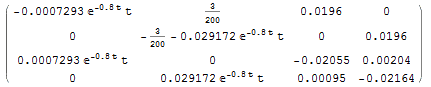# How to solve linear first order variable coefficient systems?

• robijnix

#### robijnix

1. how do i solve
X'(t)=A(t)X(t)

## Homework Equations

I know of this equation:
$x(t) = x_0 \exp\left( \int_{t_0}^t f(\xi) \, d\xi \right)$

but i think that I'm using it wrong.

## The Attempt at a Solution

i have the following mathematica code:
A=x = MatrixExp[Integrate[A, {t, 0, s}]].{1, 0,0,0};
Plot[x[] + x[], {s, 0, 500}]

the problem is, that the result i get it not the same as when i numerically plot it with matlab, which leads me to believe that I'm doing something wrong.

any kind of help would be greatly appreciated,

Thanks

Last edited:
Never mind i found out the problem.

my matrix A doesn't statisfy A(t1)A(t2)=A(t2)A(t1), in which case the above formula is not valid